基于计算机视觉的棋盘图像识别

2020/12/24 17:00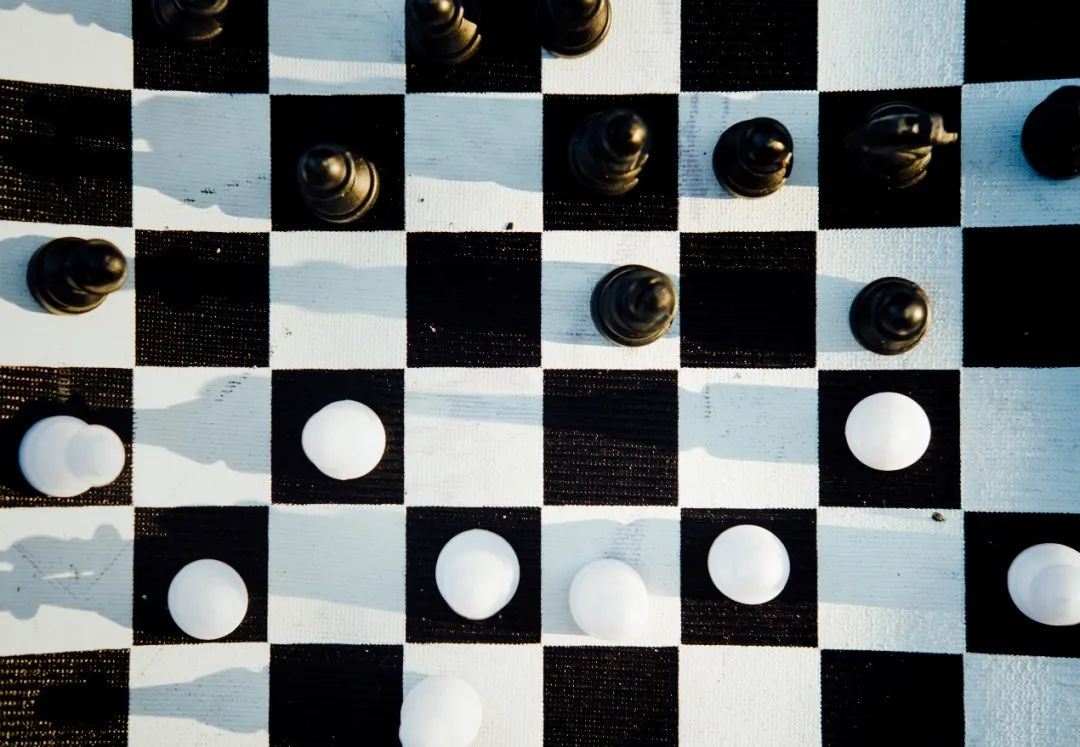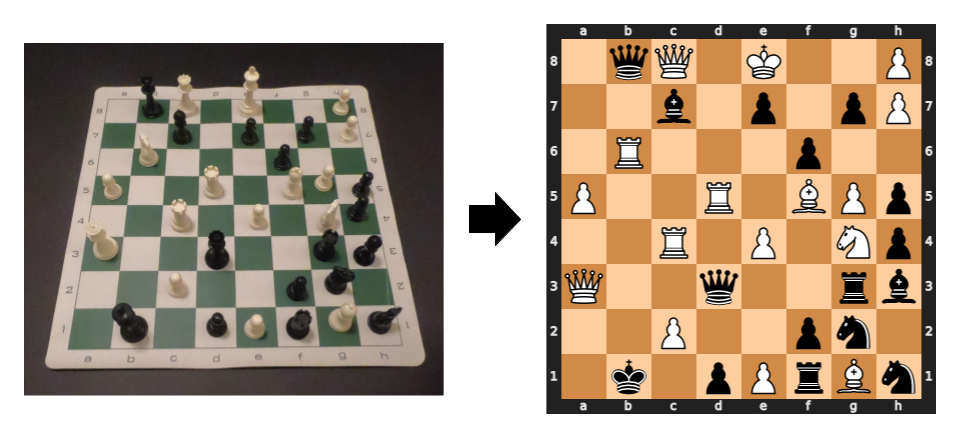（左）实时摄像机进给的帧和棋盘的（右）二维图像

01. 数据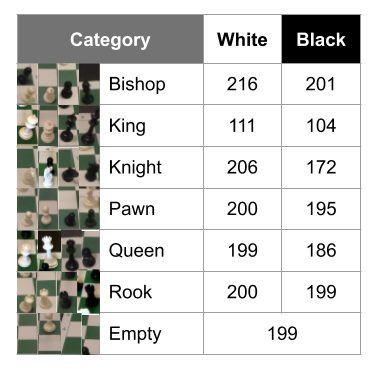02. 棋盘检测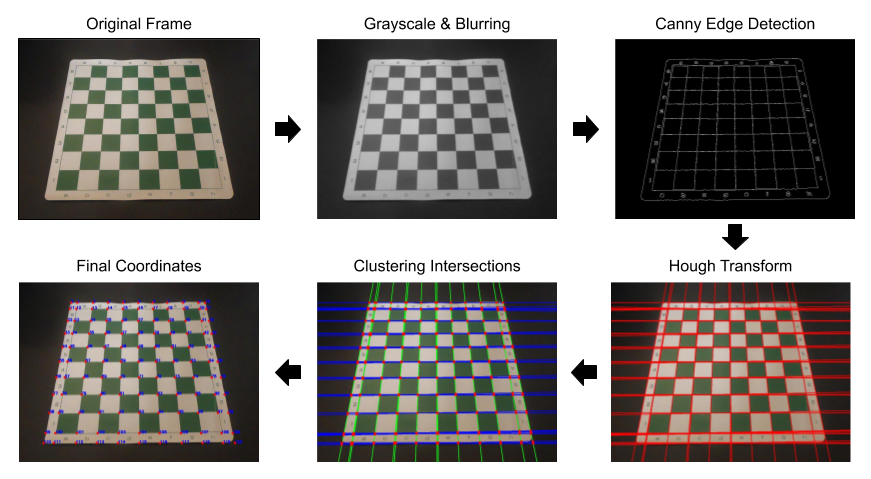03. 棋盘分类

from keras.preprocessing.image import ImageDataGeneratordatagen = ImageDataGenerator(        rotation_range=5,        rescale=1./255,        horizontal_flip=True,        fill_mode='nearest')test_datagen = ImageDataGenerator(rescale=1./255)train_gen = datagen.flow_from_directory(    folder + '/train',    target_size = image_size,    batch_size = batch_size,    class_mode = 'categorical',    color_mode = 'rgb',    shuffle=True)test_gen = test_datagen.flow_from_directory(    folder + '/test',    target_size = image_size,    batch_size = batch_size,    class_mode = 'categorical',    color_mode = 'rgb',    shuffle=False)

1.从先前训练的模型（VGG16）中获取图层。

from keras.applications.vgg16 import VGG16model = VGG16(weights='imagenet')model.summary()

2.冻结他们，以避免破坏他们在训练回合中包含的任何信息。

3.在冻结层的顶部添加了新的可训练层。

from keras.models import Sequentialfrom keras.layers import Dense, Conv2D, MaxPooling2D, Flattenfrom keras.models import Modelbase_model = VGG16(weights='imagenet', include_top=False, input_shape=(224,224,3))  # Freeze convolutional layers from VGG16for layer in base_model.layers:    layer.trainable = False# Establish new fully connected blockx = base_model.outputx = Flatten()(x) x = Dense(500, activation='relu')(x) x = Dense(500, activation='relu')(x)predictions = Dense(13, activation='softmax')(x)# This is the model we will trainmodel = Model(inputs=base_model.input, outputs=predictions)model.compile(optimizer='adam', loss='categorical_crossentropy', metrics=['categorical_accuracy'])

4.在自定义数据集上训练新层。

epochs = 10history = model.fit(    train_gen,     epochs=epochs,    verbose = 1,    validation_data=test_gen)model.save_weights('model_VGG16.h5')

04. 结果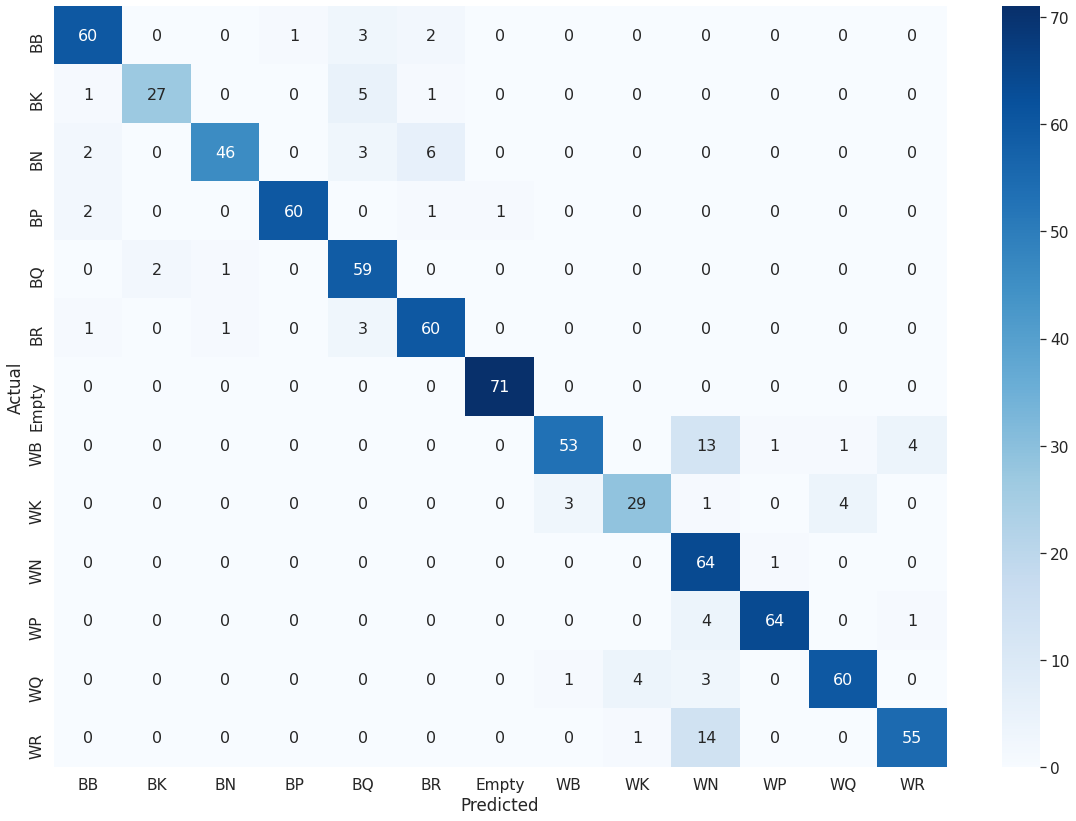05. 应用

print('Working...')        # Save the frame to be analyzed        cv2.imwrite('frame.jpeg', frame)        # Low-level CV techniques (grayscale & blur)        img, gray_blur = read_img('frame.jpeg')        # Canny algorithm        edges = canny_edge(gray_blur)        # Hough Transform        lines = hough_line(edges)        # Separate the lines into vertical and horizontal lines        h_lines, v_lines = h_v_lines(lines)        # Find and cluster the intersecting        intersection_points = line_intersections(h_lines, v_lines)        points = cluster_points(intersection_points)        # Final coordinates of the board        points = augment_points(points)        # Crop the squares of the board a organize into a sorted list        x_list = write_crop_images(img, points, 0)        img_filename_list = grab_cell_files()        img_filename_list.sort(key=natural_keys)        # Classify each square and output the board in Forsyth-Edwards Notation (FEN)        fen = classify_cells(model, img_filename_list)        # Create and save the board image from the FEN        board = fen_to_image(fen)        # Display the board in ASCII        print(board)# Display and save the chessboard image        board_image = cv2.imread('current_board.png')        cv2.imshow('current board', board_image)        print('Completed!')

AI算法与图像处理」公众号后台回复：leetcode，即可下载。每题都 runtime beats 100% 的开源好书，你值得拥有！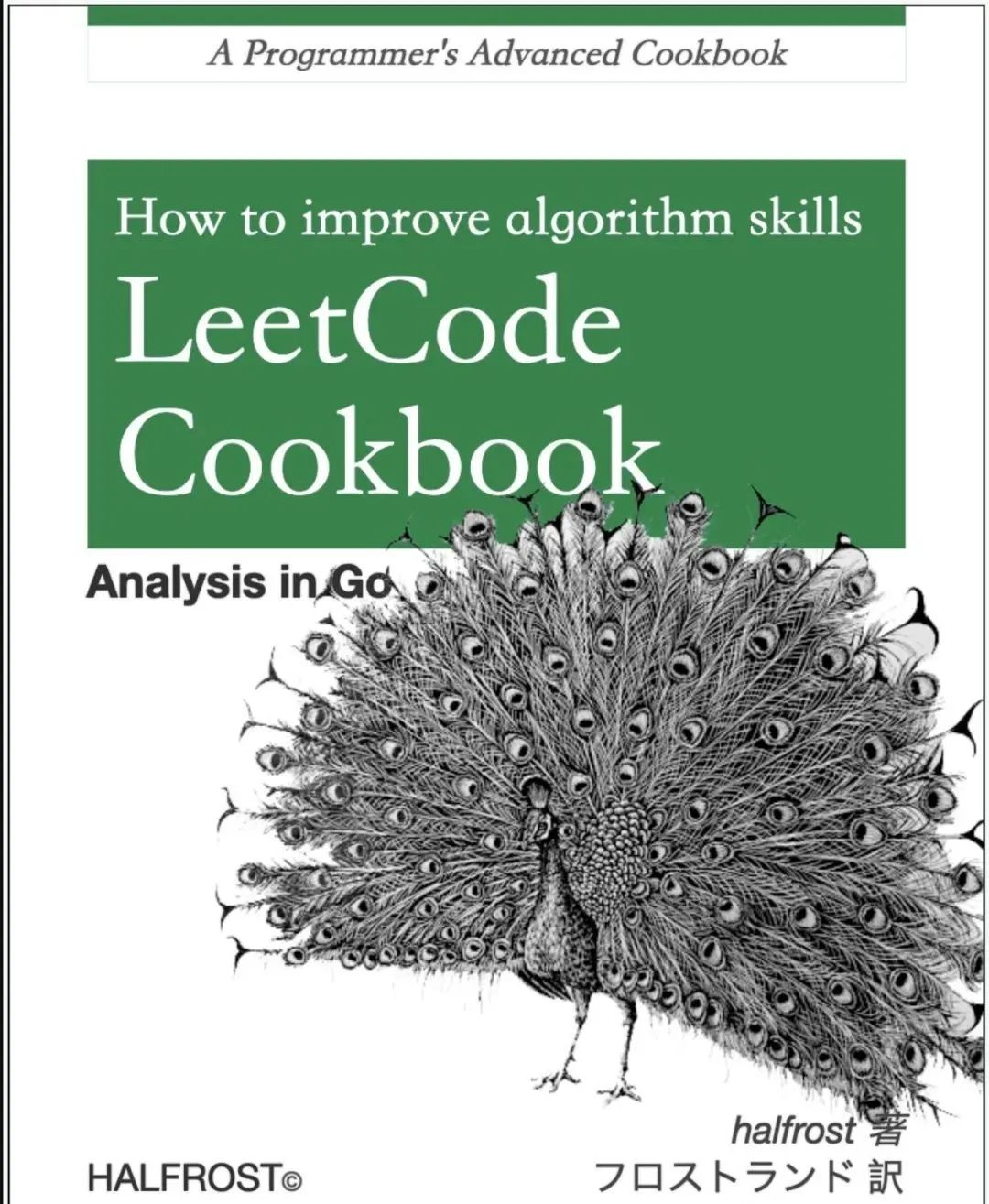AI算法与图像处公众号后台回复： CVPR2020 即可下载1467篇CVPR 2020论文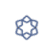1
0 收藏

作者的其它热门文章0 评论
0 收藏
1